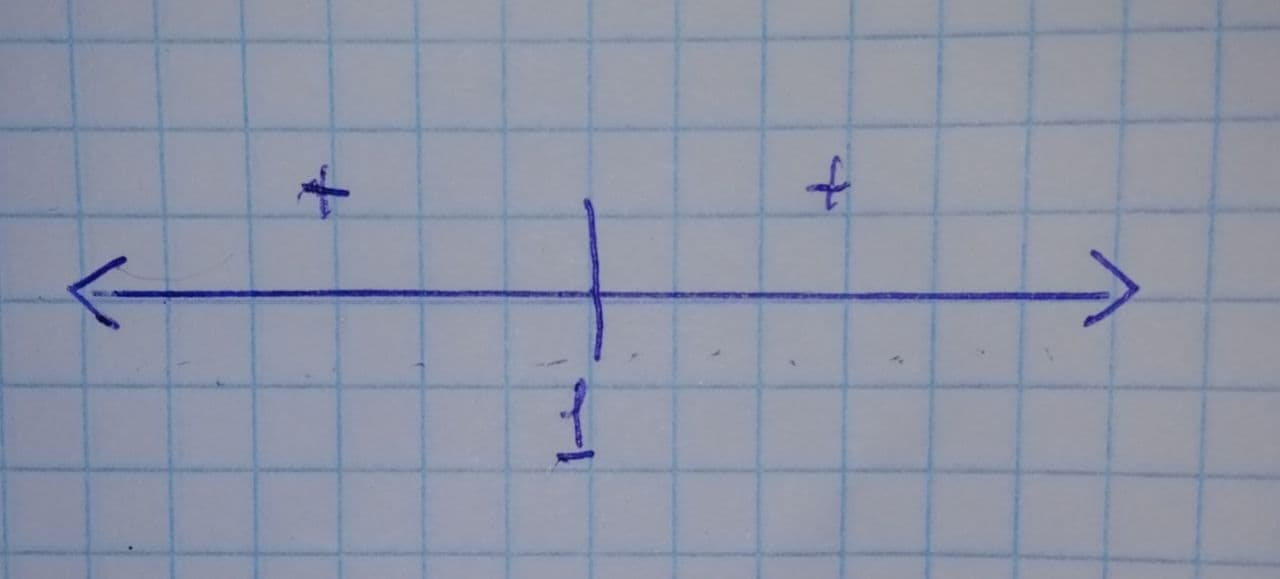philosphy111of

2021-11-26

Solve the polynomial inequality
${x}^{2}-2x+1>0$
and graph the solution set on a real number line. Express the solution set in interval notation.giskacu

Step 1
The given polynomial inequality is
${x}^{2}-2x+1>0$
To solve the inequality, find its critical points by equating it to zero.
${x}^{2}-2x+1=0$
${x}^{2}-x-x+1=0$
$x\left(x-1\right)-1\left(x-1\right)=0$
$\left(x-1\right)\left(x-1\right)=0$

Step 2
Now, make the sign analysis on Number line to determine about the inequality.
If $x<1$, then for the given polynomial the result is always positive and even if $x>1$, then also for the given polynomial the result is positive. Therefore, for all values of x except 1 ,the polynomial is positive.Answer: Hence, the interval notation for the given polynomial inequality is

Do you have a similar question?